# How to Make a Calculator in Excel for Your Toolbar

Jan 10, 2023

Are you sick and tired of trying to create formulas to do basic calculations in Microsoft Excel?

Learning how to divide numbers through Excel formulas can be a nightmare, so finding more complex equations like the square root can be almost impossible.

You might be aware already, but a calculator is built into Microsoft Excel to save you time and effort.

However, to select a calculator, you must go into your Excel ribbon every time, which can take time and effort.

This article will show you how to make a calculator in excel for your toolbar.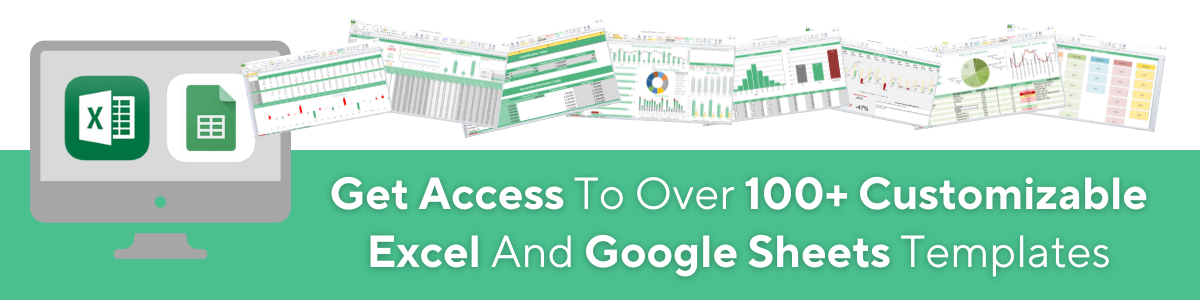1. Click on the down arrow locked in the top left-hand corner of the Excel Ribbon, located in your Excel toolbar.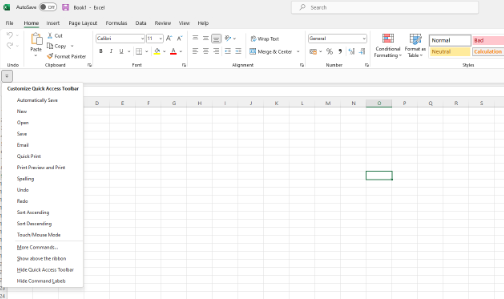1. From here, you will see a drop-down menu appear. Click the More Commands option for the list of choices.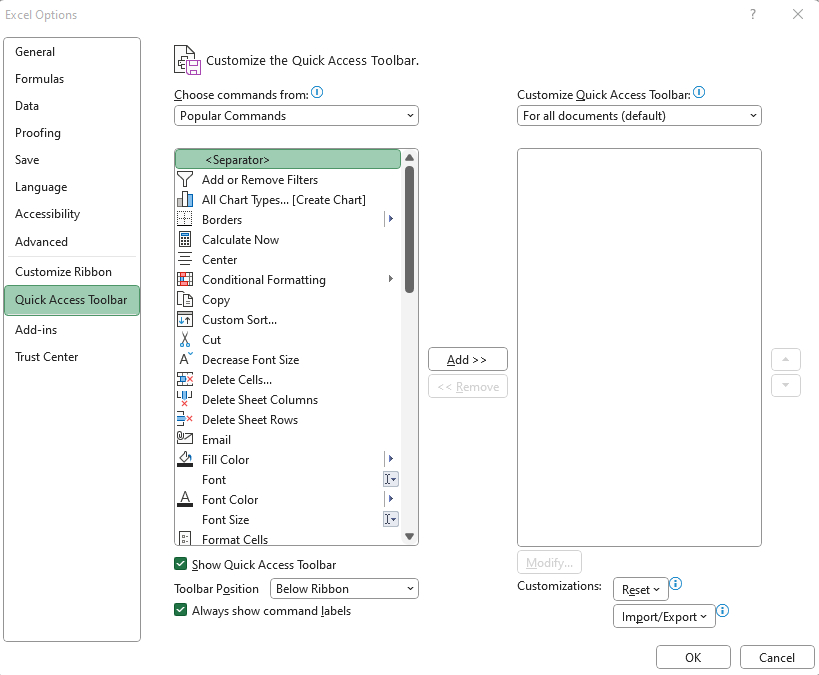1. After this, click on Commands Not in the Ribbon. This section will highlight any commands you can add to your ribbon that are not currently on your toolbar, including the calculator.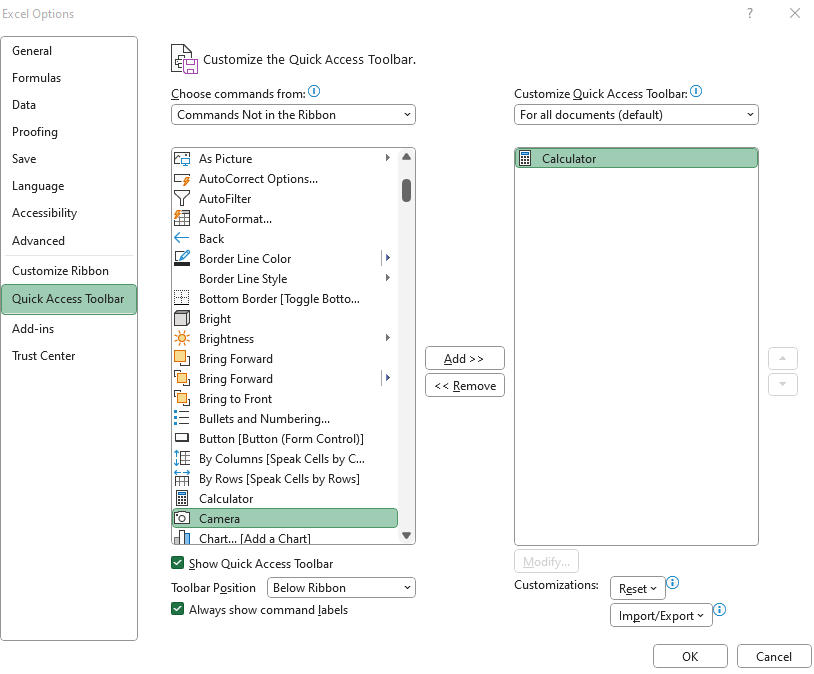1. Scroll down from the list of Commands, and choose Calculator. Select Add.

2. Once you have added the Calculator, select OK.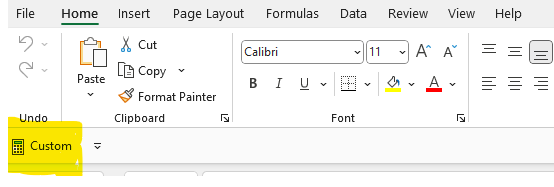If you have followed these steps correctly, the Calculator icon will appear on your Excel Quick Access toolbar.Now you have established how to implement the calculator option in Microsoft Excel. It is essential also to learn how to remove the calculator if you no longer need it.

1. Head to the top left-hand corner of your Excel Ribbon, and select the down arrow from the Microsoft Excel quick access toolbar.

2. The same drop-down menu will appear. From here, choose More Commands for the list.

3. From the Customize Quick Access Toolbar, choose Calculator and select Remove.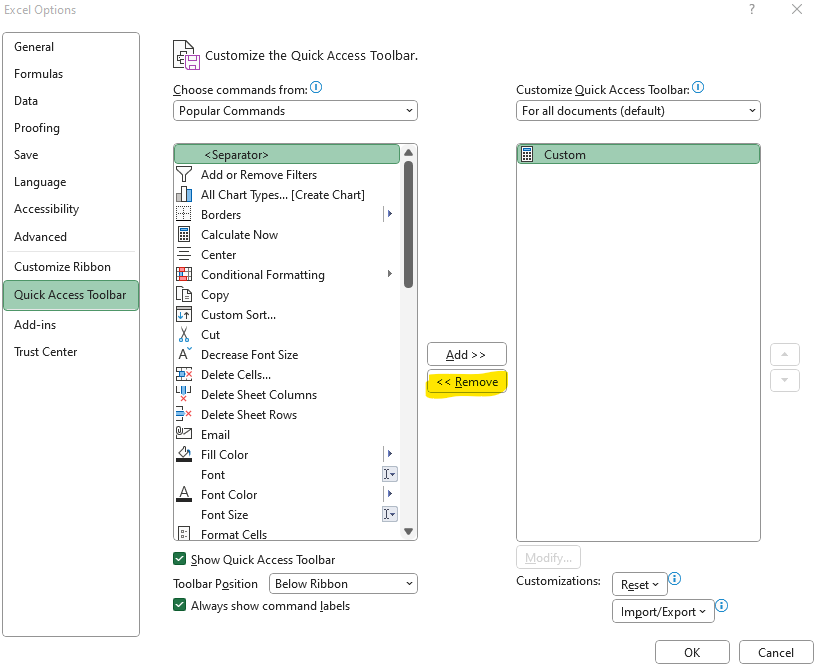1. Select OK.

## How to Use Calculator in Microsoft Excel

A calculator tool is a fantastic option for you in Microsoft Excel, as it offers a lot more functions than a simple addition and subtraction calculator.

In total, there are three excellent uses for the calculator:

Scientific: The Excel calculator is excellent for writing formulas such as trigonometric degrees, modules, and other features.

Standard: it also provides the essential functions of any calculator, letting you subtract numbers, add numbers, calculate percentages, and work with fractions.

Date Calculation: The Excel calculator is excellent for estimating the difference between two dates using the equal sign, minus sign, and plus sign.

## Final Thoughts on Using a Calculator Excel

Now you should have everything you need to add and remove a calculator in Microsoft excel. Remember to head to the top left corner, above the formula bar, to add your calendar to the quick access toolbar.

An Excel calculator can help you create simple formulas and link to cell references, making it a great addition to your quick-access toolbar.## Related Articles on MS Excel

How to Add a Trendline in Excel Online

Microsoft Excel Uses: Effective Ways to Use Microsoft Excel

Basic Microsoft Excel Formulas You NEED to Know

#### Want to Make Excel Work for You? Try out 5 Amazing Excel Templates & 5 Unique Lessons

We hate SPAM. We will never sell your information, for any reason.